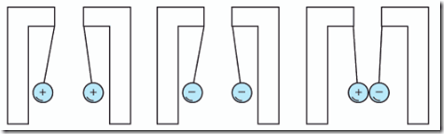Home > English > Atomic Properties

# Atomic Properties

Terms imposed on the ion in an atom or group of atoms to lose one or more electrons, resulting in a positive charge (commonly known as cations) in atomnya.Sebaliknya an atom or group of atoms get extra electrons so that the negatively charged (commonly known as the anion).

Essential properties of protons and electrons:
– The proton is 1.66 x 10-27 kg.
– The electron is 9.1096 x 10-31 kg.

– The electric charge is measured in coulombs (C), where one coulomb is equal to the amount of cargo by 6.24 x 1018 electrons.
– The charge of an electron is e = 1.602 x 10-19 Coulomb.
– The number of electrons in each atom of different objects. For example, the number of electrons in copper is 29.
– On an object, if the number of positive charge and a negative charge at the object is to be neutral.

From experiments using the electroscope, it can be seen that if there are two positive charges adjacent to happen repulsion, and so if there are two adjacent negative charge. If the positive charge adjacent to the negative charge will arise attractive force.Figure 1.8 The style of departure and force of electric attraction

The phenomenon of the electric force is factual knowledge in the world of static electricity. Electric force is meant here is repulsive and attractive force that arises at a single atom or group of atoms. The style may arise due to an excess or shortage of the number of electrons in the atom. When one positively charged atoms and there are other negatively charged atoms then occurs tensile force between the two. Conversely when both atoms are the same then occurs between the repulsive force occurring keduanya.Fenomena electric force on a charged atom would have raised a very interesting question."Tasikmalaya, Indonesia"